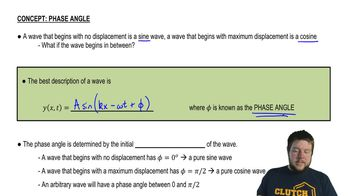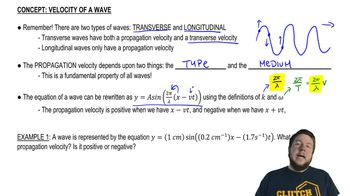Start typing, then use the up and down arrows to select an option from the list.
6:14 minutes
Problem 15a
Textbook Question

# A fellow student with a mathematical bent tells you that the wave function of a traveling wave on a thin rope is y(x, t)=2.30mm cos[(16.98 rad/m^)x+(742 rad/s)t]. Being more practical, you measure the rope to have a length of 1.35 m and a mass of 0.00338 kg. You are then asked to determine the following: (d) wave speed; (e) direction the wave is traveling;Verified Solution
This video solution was recommended by our tutors as helpful for the problem above.
31views